# Basic functions - math word problems

#### Number of problems found: 2274

• Big factorial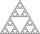How many zeros end number 116! ?
• Seven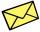Seven friends agree to send everyone a holiday card. How many postcards were sent?
• Promile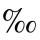Calculate the 4.6 ‰ of 199.
• Image scale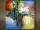The actual image dimensions are 60 cm x 80cm and has a reduced size 3 cm x 4 cm. At what scale the image was reduced?
• Percentage 1052 shorts and missed 13. Calculate percentage
• Seeds 2How many seeds germinated from 1000 pcs, when 23% no emergence?
• One sixth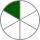How many sixths are two thirds?
• Hockey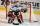Hockey match ended 8:2. How many different matches could be?
• Squares ratioThe first square has a side length of a = 6 cm. The second square has a circumference of 6 dm. Calculate the proportions of the perimeters and the proportions of the contents of these squares? (Write the ratio in the basic form). (Perimeter = 4 * a, conte
• Perctentages35% of what number is 35?
• Powers 3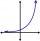2 to the power of n divided by 4 to the power of -3 equal 4. What is the vaule of n?
• Insect spraying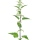In 600 ml of spraying is 5 ml of an active ingredient. How much is it per miles?
• Subtraction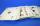How many times you can subtract the number 4 from the number 64?
• SequenceCalculate what member of the sequence specified by ? has value 86.
• Harmonic 4Harmonic mean of -6 and 5.
• Possible combinations - word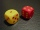How many ways can the letters F, A, I, R be arranged?
• LogarithmDetermine the number whose decimal logarithm is -3.8.
• Committees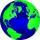How many different committees of 2 people can be formed from a class of 21 students?
• Reciprocal equation 2Solve this equation: x + 5/x - 6 = 4/11
• Simply equationSolve this equation for x: ?

Do you have an interesting mathematical word problem that you can't solve it? Submit a math problem, and we can try to solve it.

We will send a solution to your e-mail address. Solved examples are also published here. Please enter the e-mail correctly and check whether you don't have a full mailbox.

Please do not submit problems from current active competitions such as Mathematical Olympiad, correspondence seminars etc...